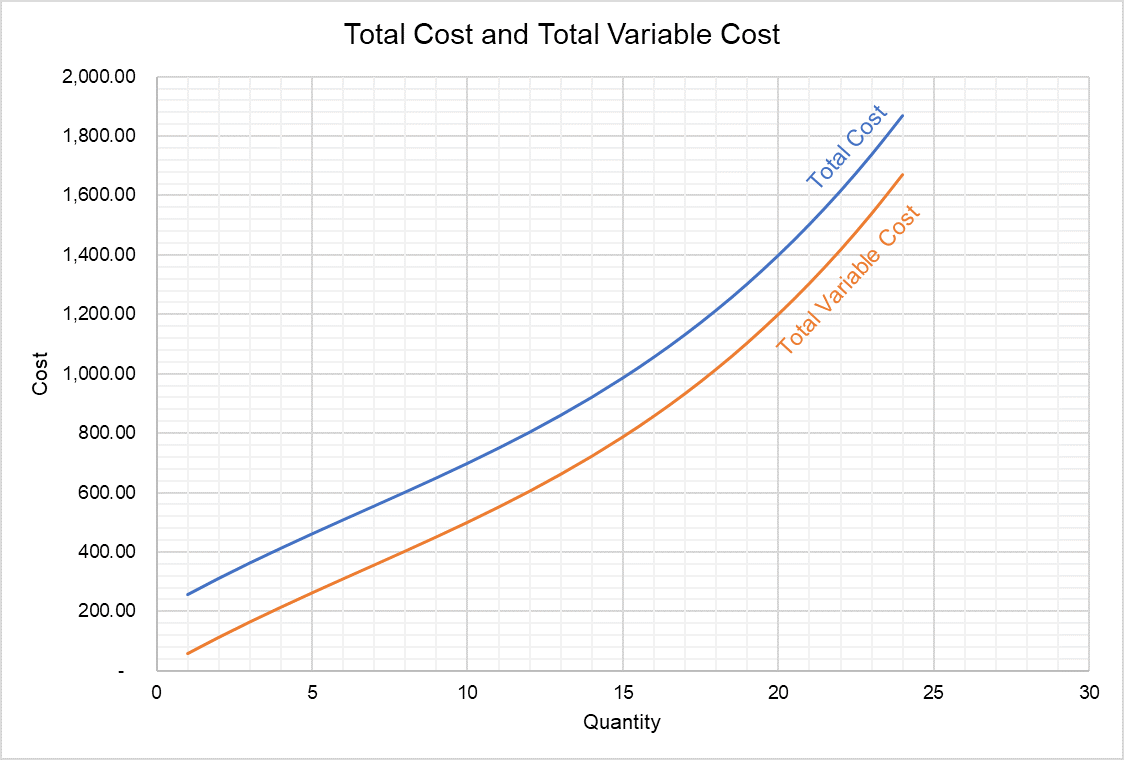# Cost Functions

A cost function is a mathematical relationship between cost and output. It tells how costs change in response to changes in output.

Even though relationship between a firm’s costs and output can be studies using cost tables (which show total cost, total variable cost and marginal cost for each unit) or graphs which plot different cost curves, a cost function is the most compact and direct method of encapsulating information about a firm’s costs.

Cost functions typically have cost as a dependent variable and output i.e. quantity as an independent variable. Such cost functions do not account for any changes in cost of inputs because they assume fixed input prices.

## Types of Cost Functions

Typical cost functions are either linear, quadratic and cubic.

A linear cost function is such that exponent of quantity is 1. It is appropriate only for cost structures in which marginal cost is constant.

A quadratic cost function, on the other hand, has 2 as exponent of output. It represents a cost structure where average variable cost is U-shaped.

A cubic cost function allows for a U-shaped marginal cost curve. The cost function in the example below is a cubic cost function.

Total cost function is the most fundamental output-cost relationship because functions for other costs such as variable cost, average variable cost and marginal cost, etc. can be derived from the total cost function.

## Example

Imagine you work at a firm whose total cost (TC) function is as follows:

$$\text{TC}\ =\ \text{0.1Q}^\text{3}-\ \text{2Q}^\text{2}+\text{60Q}+\text{200}\$$

Average total cost function can be derived by dividing the total cost function by Q:

$$\text{ATC}\ =\ \frac{\text{TC}}{\text{Q}}=\text{0.1Q}^\text{2}-\ \text{2Q}+\text{60}+\frac{\text{200}}{\text{Q}}\$$

The constant value in a total cost function represent the total fixed cost. Function for total variable cost can be arrived at by subtracting the constant value from the total cost function:

$$\text{VC}=\text{TC}\ -\ \text{FC}\$$

$$\text{VC}=\ \text{0.1Q}^\text{3}-\ \text{2Q}^\text{2}+\text{60Q}$$

Average variable cost function equals total variable cost divided by Q:

$$\text{AVC}=\frac{\text{VC}}{\text{Q}}=\ \text{0.1Q}^\text{2}-\ \text{2Q}+\text{60}$$

Marginal cost equals the slope of the total cost curve which in turn equals the first derivative of the total cost function.

$${\text{MC}} _ \text{Q}=\frac{\text{dTC}}{\text{dQ}}\ =\ \text{0.3Q}^\text{2}-\ \text{4Q}+\text{60}\$$

Cost functions can be used to create cost tables and cost curves. By plugging different quantity levels in the cost functions determined above, we can create a cost table which can be used to plot the cost curves.

The total cost and total variable cost curves represented by functions discussed above give us the following graph:Since the total cost function is a cubic-function, the average variable cost curve and the marginal cost curve are U-shaped as shown below.